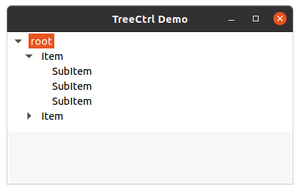Related Articles

# wxPython – ExpandAllChildren() method in wx.TreeCtrl

• Last Updated : 25 Nov, 2020

In this article, we are going to learn about ExpandAllChildren() method associated with wx.TreeCtrl class of wxPython. ExpandAllChildren() method is used in order to expand all the nodes that comes under a particular item that is passed as a parameter in the method. Basically, this method expands the given item and all its children recursively.

This function takes the tree node item as a parameter to whom we want to expand recursively.

Attention geek! Strengthen your foundations with the Python Programming Foundation Course and learn the basics.

To begin with, your interview preparations Enhance your Data Structures concepts with the Python DS Course. And to begin with your Machine Learning Journey, join the Machine Learning - Basic Level Course

Syntax:  wx.TreeCtrl.ExpandAllChildren(self,i tem)

Parameters:

Code Example:

## Python

 `import` `wx `` ` ` ` `class` `MyTree(wx.TreeCtrl): `` ` `    ``def` `__init__(``self``, parent, ``id``, pos, size, style): ``        ``wx.TreeCtrl.__init__(``self``, parent, ``id``, pos, size, style) `` ` ` ` `class` `TreePanel(wx.Panel): `` ` `    ``def` `__init__(``self``, parent): ``        ``wx.Panel.__init__(``self``, parent) ``         ` `        ``# create tree control in window ``        ``self``.tree ``=` `MyTree(``self``, wx.ID_ANY, wx.DefaultPosition, ``                           ``wx.DefaultSize, wx.TR_HAS_BUTTONS) ``         ` `        ``# CREATE TREE ROOT ``        ``self``.root ``=` `self``.tree.AddRoot(``'root'``) ``        ``self``.tree.SetPyData(``self``.root, (``'key'``, ``'value'``)) `` ` `        ``# add item to root ``        ``item ``=` `self``.tree.AppendItem(``self``.root, ``"Item"``) ``        ``item2 ``=` `self``.tree.AppendItem(``self``.root, ``"Item"``) ``        ``item3 ``=` `self``.tree.AppendItem(item, ``"SubItem"``)``        ``item4 ``=` `self``.tree.AppendItem(item, ``"SubItem"``)``        ``item5 ``=` `self``.tree.AppendItem(item2, ``"SubItem"``)``        ``item6 ``=` `self``.tree.AppendItem(item, ``"SubItem"``)`` ` `        ``# expand root node``        ``self``.tree.Expand(``self``.root)`` ` `        ``# expand all nodes of the tree``        ``self``.tree.ExpandAllChildren(item) ``         ` `        ``sizer ``=` `wx.BoxSizer(wx.VERTICAL) ``        ``sizer.Add(``self``.tree, ``0``, wx.EXPAND) ``        ``self``.SetSizer(sizer) `` ` ` ` `class` `MainFrame(wx.Frame): `` ` `    ``def` `__init__(``self``): ``        ``wx.Frame.__init__(``self``, parent ``=` `None``, title ``=``'TreeCtrl Demo'``) ``        ``panel ``=` `TreePanel(``self``) ``        ``self``.Show() `` ` ` ` `if` `__name__ ``=``=` `'__main__'``: ``    ``app ``=` `wx.App(redirect ``=` `False``) ``    ``frame ``=` `MainFrame() ``    ``app.MainLoop()`

Output:My Personal Notes arrow_drop_up